# ISEE Middle Level Math : How to find the probability of an outcome

## Example Questions

### Example Question #822 : Isee Middle Level (Grades 7 8) Mathematics Achievement

There are 4 green marbles, 5 yellow marbles, and 3 red marbles in a bag. If one is picked out of the bag at random, what is the probability that it will be a red marble?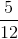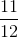Explanation:

To find probability, you have to consider part over whole. The total number of marbles is 12. Then, identify how many red marbles there are (3). From there, you can make a fraction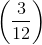, which can be simplified to.

### Example Question #11 : Develop And Compare Probability Models And Find Probabilities Of Events: Ccss.Math.Content.7.Sp.C.7

A pair of fair dice are thrown. Which of these events has probability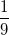?

Both dice show the same number

The sum of the dice is divisible by 6

The sum of the dice is 10

The sum of the dice is 5

The sum of the dice is 4.

The sum of the dice is 5

Explanation:

One-ninth of the 36 possible rolls is 4, so we are looking for an outcome that can happen four ways.

There are 6 ways that both dice can show the same number - double 1, double 2, and so forth.

There are 3 ways to roll a sum of 10: (4,6), (5,5), and (6,4).

There are 6 ways to roll a sum divisible by 6: (1,5), (2,4), (3,3), (4,2), and (5,1) all yield 6, and (6,6) yields 12.

There are 4 ways to roll a sum of 5: (1,4), (2,3), (3,2), and (4,1).

There are 3 ways to roll a sum of 4: (1,3), (2,2), (3,1).

The correct choice is the event that the sum of the dice is 5.

### Example Question #11 : How To Find The Probability Of An Outcome

Gina has a bag of 12 marbles. The bag is half black marbles and half white marbles. If Gina picks out two random marbles, and both are white, what is the percent chance that the next marble she picks will also be white?

40 percent

50 percent

60 percent

20 percent

40 percent

Explanation:

Given that in Gina’s bag of 12 marbles, half of them are black and half are white, this means that there are 6 black marbles and 6 white marbles. However, because she already picked out 2 white marbles, this means that there are now only 4 out of 10 white marbles left. 4 out of 10 converts to 40 percent, which is therefore the correct answer.

### Example Question #821 : Isee Middle Level (Grades 7 8) Mathematics Achievement

10% of puppies are born with spots. Of those, 50% have black spots. What is the percentage of puppies who have black spots?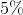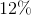Explanation:

If 10 percent of puppies are born with spots, and of those, 50 percent have black spots, then the total percent of puppies who have spots AND the color of the spots is black can be found by multiplying the two percentages by one another: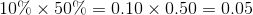0.05 is the equivalent of 5 percent, the correct answer.

### Example Question #831 : Isee Middle Level (Grades 7 8) Mathematics Achievement

Jenny entered a raffle with 9 other participants. The raffle ticket numbers are:

288, 289, 290, 291, 292, 292, 294, 295, 296, 297

Jenny's ticket is 288. The raffler begins to announce the winning ticket. The first digits are 2 and 8. He is about to announce the third digit.

What are the chances that Jenny won?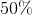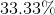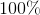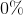Explanation:

There are 10 total participants in the raffle.

288, 289, 290, 291, 292, 292, 294, 295, 296, 297

Only 2 participants have tickets with numbers that beging in 2 and 8.; therefore, Jenny has a 50 percent chance of holding the winning raffle ticket.

The winner is either 288 or 289, leaving Jenny a 50 percent chance.

### Example Question #781 : Grade 7

At a party, there are 5 girls and 5 boys. There is a raffle, and each girl gets twice as many raffle tickets as each boy. Tracy is one of the girls at the party. What is the chance of her winning the raffle if each boy gets 7 raffle tickets?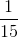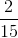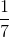Explanation:

If each boy gets 7 raffle tickets, that means that each girl gets 14 raffle tickets.

Therefore, Tracy will have 14 raffle tickets, and the total number of raffle tickets will be equal to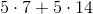Thus, Tracy's chance of winning will be equal to: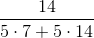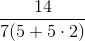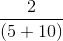### Example Question #11 : Probability

Set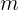consists of the numbers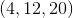and setconsists of the numbers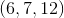. If a number,is chosen at random from set, and another number,is chosen at random from set, what is the probability that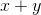will be equal to an odd number?Explanation:

Whenever an even number is added to an odd number, the result is odd. Whenever an even number is added to an even number, the result is even.

Given that all the numbers in setare even, there is a 100 percent chance of an even number being selected; however in set, one of the three numbers is odd, so there is a one third chance of an odd number being selected.

Given that the sum of an even number and an odd number is odd, there is a probability ofthat the sum of a number randomly selected from setand from setwil be an odd number.

### Example Question #11 : Probability

Setconsists of the numbers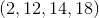and set s consists of the numbers. If a number,is chosen at random from set, and another number,is chosen at random from set, what is the probability that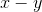will be a negative number?Explanation:

The only way in which a negative number would be acheived by subtractingis ifwas equal to 2, and any number was selected forTherefore, the chance ofresulting in a negative number would be equal to the odds of selecting 2 from set. Given that there are four numbers in set, there is a one fourth chance.

Thus, the correct answer is.

### Example Question #12 : How To Find The Probability Of An Outcome

Setconsists of the numbers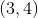. Set n consists of the numbers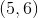. If a number is randomly selected from setand multiplied by a number randomly selected from set, what is the chance the product will be?Explanation:

The only way that a product ofwill be acheived is if the numberis selected from set, and the numberis selected from setThere is a one half chance of the number 4 being selected and a one half chance of the number 6 being selected, given that each set contains two numbers.

Therefore, the probability of both these number being chosen is: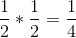### Example Question #5 : How To Find The Probability Of An Outcome

If Mark flips a coin and then rolls a die, what are the odds that the coin will be heads and that the die will land on a multiple of 3?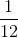Explanation:

If Mark flips a coin, the chance that it will land on heads is. On a die, there are 2 out of 6 numbers that are a multiple of 3 (3 and 6); therefore, there is achance that the dice will be a multiple of 3.

The probability that the coin will land on heads and that the dice will be a multiple of 3 is: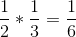### All ISEE Middle Level Math Resources